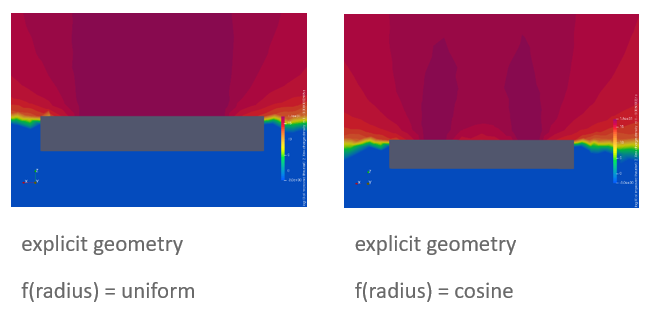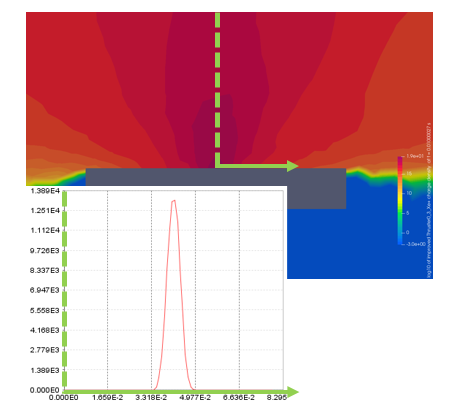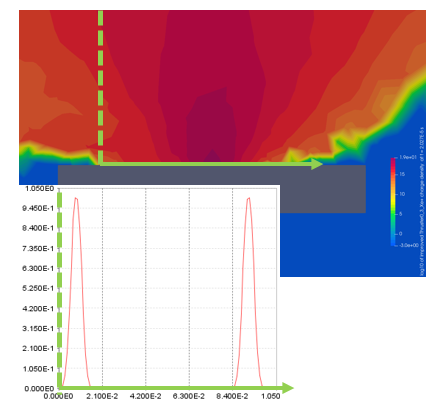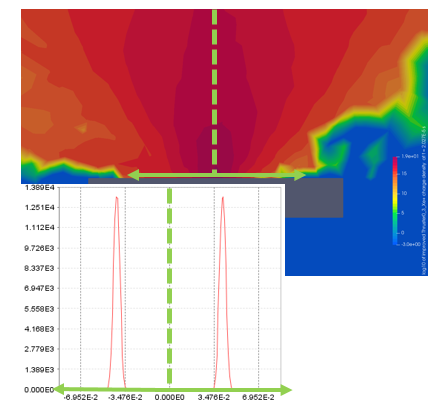# Define a thruster f(radius) distribution

Hello everyone,

I am working on simulating the plume of a SPT-100 and for this I define the 3 thruster distributions in SPIS (energy, angle and radial) assuming:

• energy distribution the kinetic energy of the neutrals, ions and doubly charged ions respectively
• angle distribution the angle between total velocity vector and the thruster axis
• radial distribution the number of particles along the thruster exit

Using the distribution (uniform or cosine) provided results in the following Xe+ charge density plots:However when I define a 1D-array with an own particle density I always obtain a plume coming out from the centre of the thruster exit. The following 3 plots show in the foreground the radial distribution (probability over radius) and in the background the resulting Xe+ charge density.

So my question would be how the radial distribution is meant to be defined?